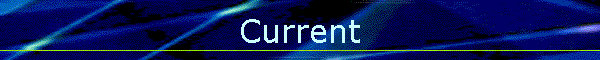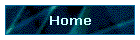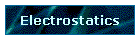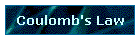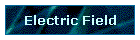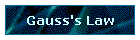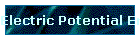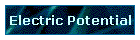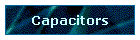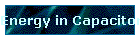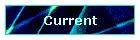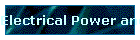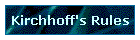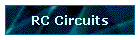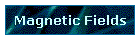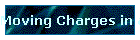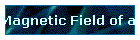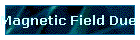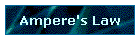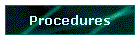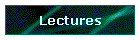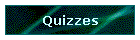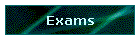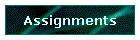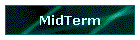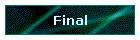Phys 4, Section 2                                                                 Feb 4, 2002   Current Current is the motion of charge:            I = dq/dt where I is measured in amperes (A). The direction of the current is always the direction of positive charge motion. This means that electron flow is always opposite to the direction of current flow. Assume an electric field E is established across a straight piece of wire of cross-section A. Under the influence of the electric field charged particles in the (conducting) wire will start drifting through the field. Assume the average drift velocity of the charges is vd and the density of charges is n. Then the charge dq moving past a particular cross-section of the wire is             dq = q(nAvddt) = nqvdAdt and the current I is given by             I = dq/dt = nqvdA. The current density (J) is defined as J = I/A = n|q|vd. The drift velocity is surprisingly low. Assume a wire of diameter 1 mm carries a current of 2.0 A. If the density of free electrons in the wire is 8.5 × 1028 electrons per cubic meter, the drift velocity is only about 0.1 mm/s and an electron would require almost 2 hours to travel one meter down the wire. Resistance Resistivity ρ is the ratio of the magnitudes of electric field E and current density J:             ρ = E/J with units (V/m)(A/m2) = (V/A)٠m = Ω٠m     where Ω = V/A is an ohm. If a wire has uniform cross-section A and length L, with resistivity ρ and a voltage V across the ends of the wire, then             E = ρJ            V/L = ρI/A            V = (ρL/A)I define resistance R = ρL/A Ohm's Law Resistance is the ratio of the potential across a material to the current through the material.             R = V/I              (Ohm's Law) R is called the resistance of a particular object measured between two points. Resistivity ρ is a characteristic of a material, while resistance R is a characteristic of a specific sample of that material, with size and geometry figured in. Although R is generally considered a constant for a particular resistor, R generally varies with temperature, with a proportionality constant α dependent on the material:             dR/dT = αR   Last modified on February 11, 2002